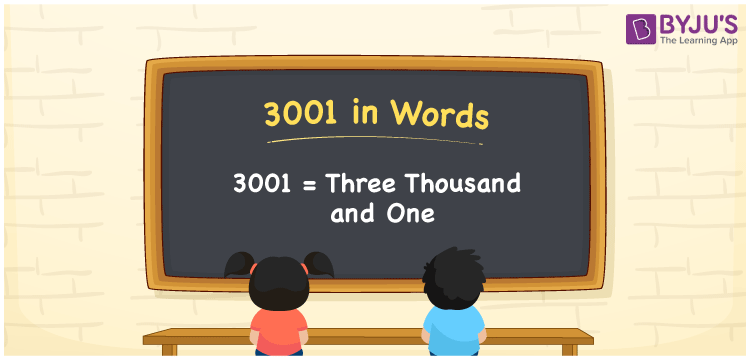# 3001 in Words

We can write 3001 in words as Three Thousand and One. Suppose you sewing machine bought a new fan that costs Rs. 3001, then you could say that “ I bought a sewing machine for Three Thousand and One rupees”. In this article, you will learn how to convert the number 3001 into words in an exciting way. The number 3001 is used in expressions that relate to money, days, distance, length, weight and so on.

 3001 in words Three Thousand and One Three Thousand and One in Numbers 3001

## 3001 in English Words

We generally write numbers in words using the English alphabet. Thus, we can read 3001 in English as “Three Thousand and One”.## How to Write 3001 in Words?

In this section, you will learn how to write the number 3001 in words. The given number 3001 has four digits, so we should make a place value chart that represents the place value for all these four digits. The order of place values of digits in a number is in accordance with the Indian numbering system, such as:

• Ones
• Tens
• Hundreds
• Thousands
• Ten-thousands
• Hundred-thousands or Lakhs

This can be done as follows.

 Thousands Hundreds Tens Ones 3 0 0 1

Thus, we can write the expanded form as:

3 × Thousand + 0 × Hundred + 0 × Ten + 1 × One

= 3 × 1000 + 0 × 100 + 0 × 10 + 1 × 1

= 3001

= One thousand five hundred

3001 is a natural number that precedes 3000 and succeeds 3002.

3001 in words – Three Thousand and One

Is 3001 an odd number? – Yes

Is 3001 an even number? – No

Is 3001 a perfect square number? – No

Is 3001 a perfect cube number? – No

Is 3001 a prime number? – Yes

Is 3001 a composite number? – No

## Frequently Asked Questions on 3001 in Words

Q1

### How do you write 3001 in English?

We can write 3001 in English words as “Three Thousand and One”.
Q2

### How do you write 3001 in words for a cheque?

For a cheque, we generally write 3001 in words as Three Thousand and One rupees only.
Q3

### What number is 3001 in words?

The number 3001 is Three Thousand and One in words.